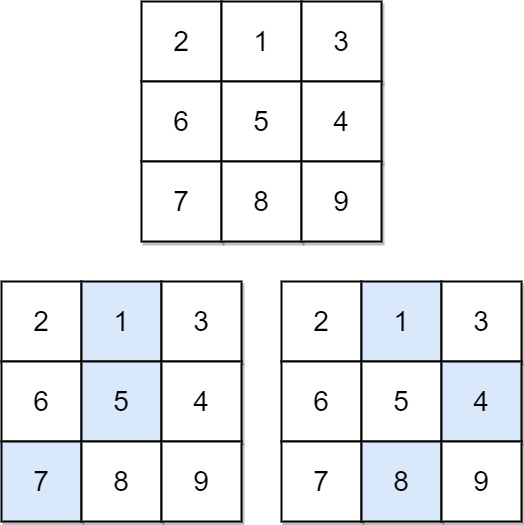604. Minimum Falling Path Sum

0

Medium

Given a square matrix of integers with dimensions n x n, find the minimum sum of any falling path through the matrix. A falling path starts at any element in the first row and chooses the element in the next row that is either directly below or diagonally left/right. Specifically, the next element from position (row, col) will be (row + 1, col - 1), (row + 1, col), or (row + 1, col + 1).Input Format

The first line contains the value of n, the number of rows and columns in the matrix. The next n lines contain n space-separated integers representing the elements of the matrix.

Output Format

Print the minimum sum of any falling path through the matrix.

Example

Input

3 2 1 3 6 5 4 7 8 9

Output

13

Constraints

n == matrix.length == matrix[i].length
1 <= n <= 100
-100 <= matrix[i][j] <= 100